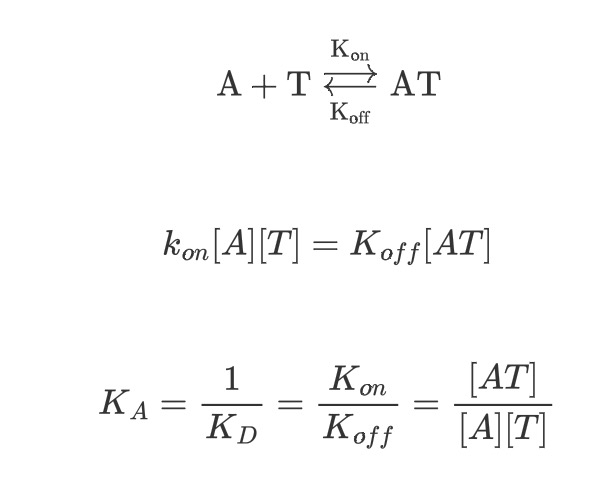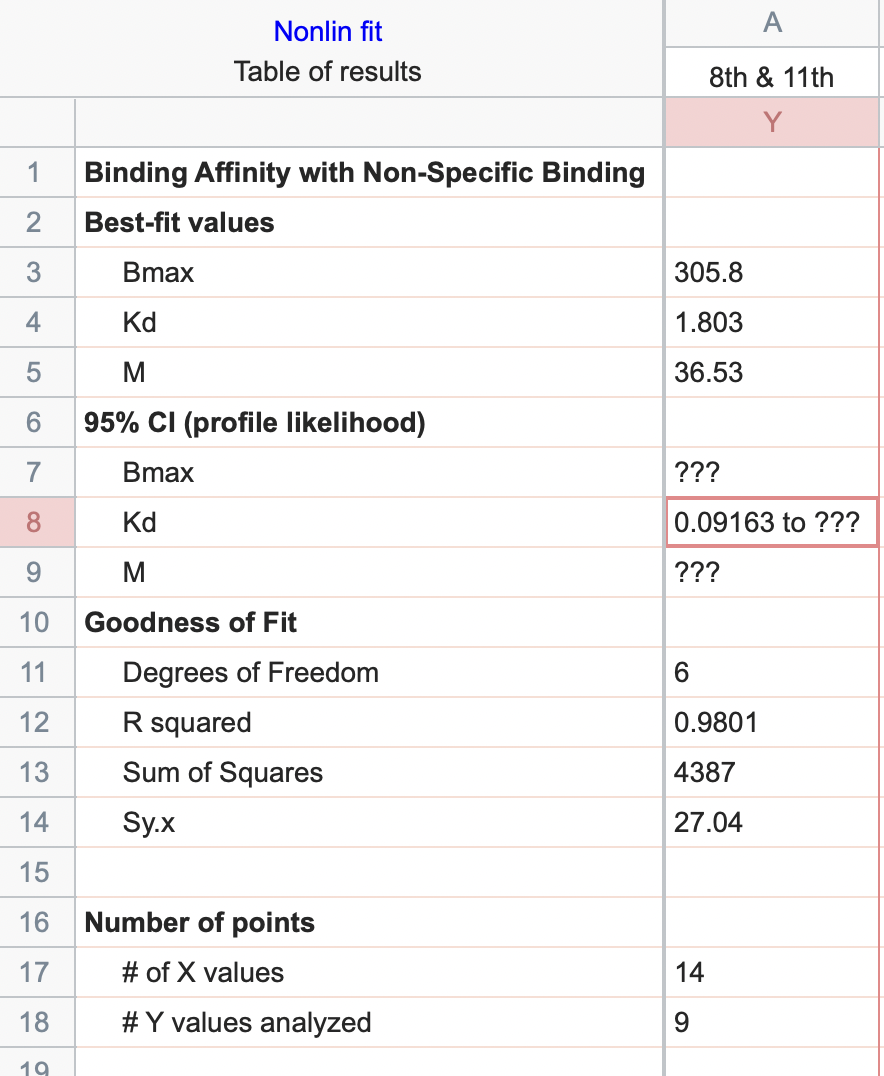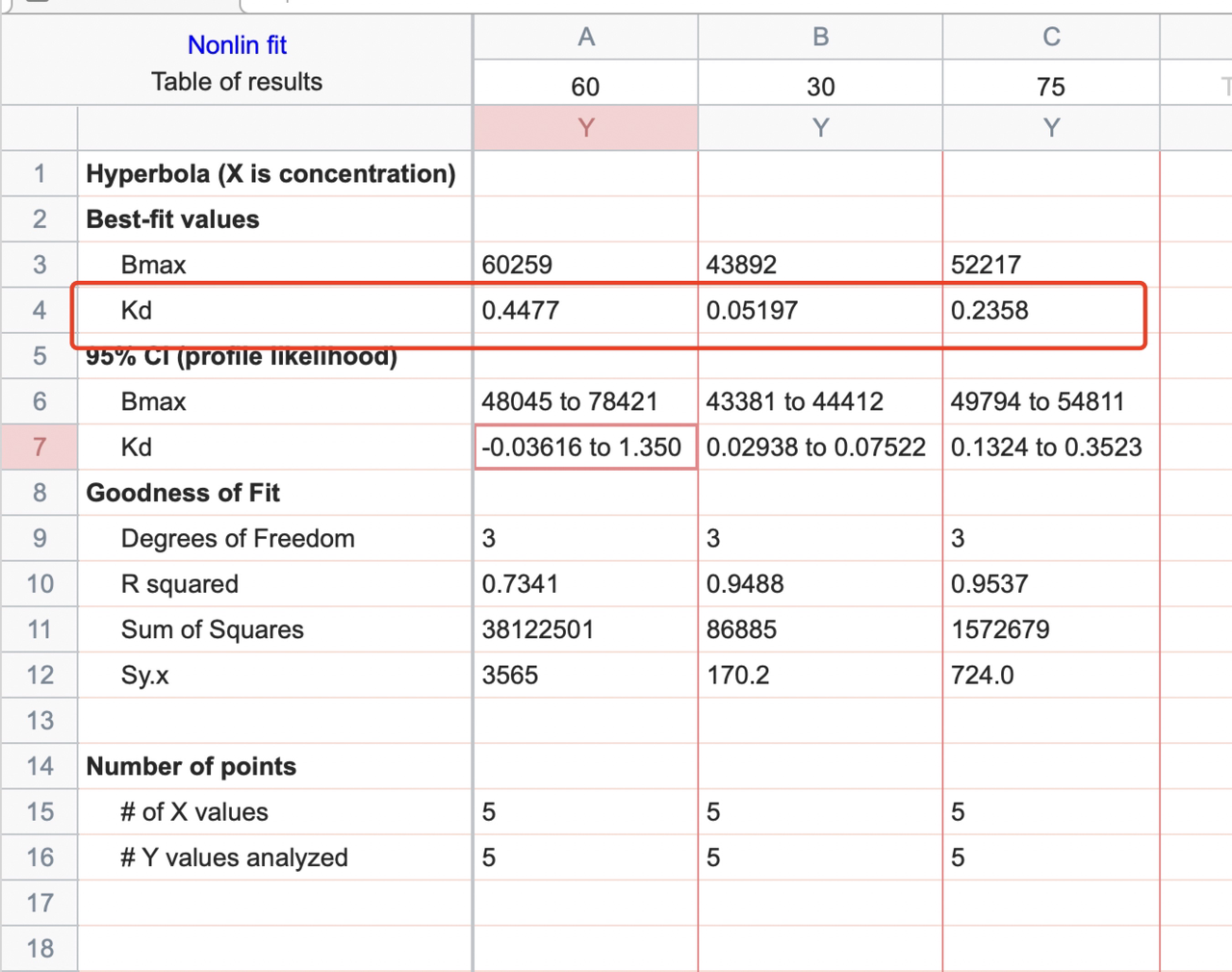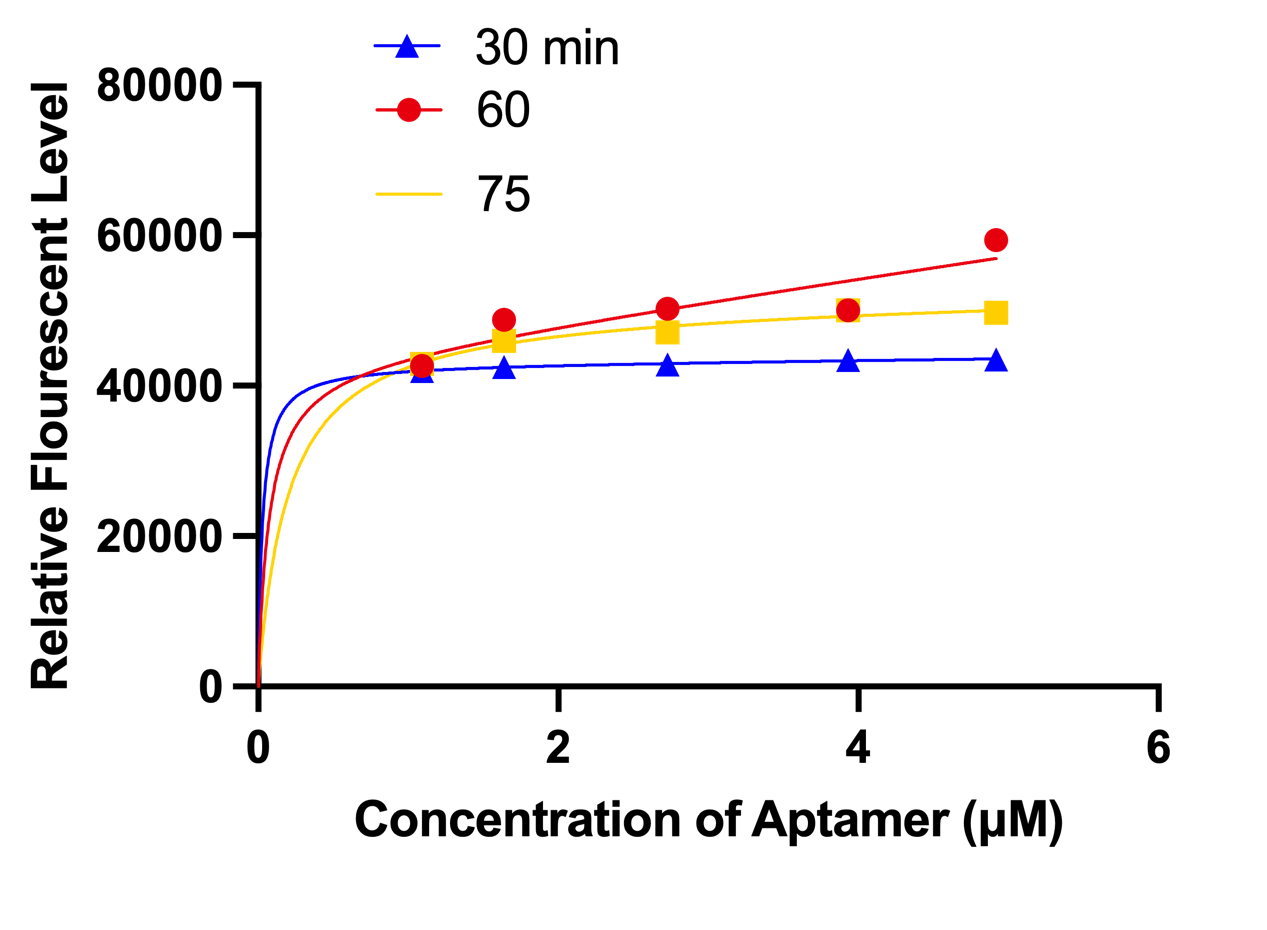# Team:GreatBay SCIE/Dissociation constant<!DOCTYPE html>
Dissociation constant

At first we planned to calculate Kd which can be derived from Hill equationBut soon we found that it is impossible to calculate in this way as the seller of the ELISA kit can't tell us the concentration of the aptamer so we had to find another way.

This equation is derived from Michaelis–Menten kinetics

$$V=V_{max}\frac{[P]}{K_M\times{[P]}}$$

With slightly modification, we can obtain the following equation

$$Y=\frac{B_{max}\times X}{K_d+X}+M\times X$$

Where Bmax is the maximum specific binding in the same units as Y.

Kd is the equilibrium dissociation constant, in the same units as X. It is the radio-ligand concentration needed to achieve a half-maximum binding at equilibrium.

MX is the slope of nonspecific binding in Y units divided by X units.

By plotting the data into the prism and doing the following steps, the system can calculate automatically

Due to the aptamer having the ability to bind with a non-specific site, thus we can add MX into the equation which leads to an increase in the affinity of the aptamer.

Using the data from the Aptamer experiments we can evaluate that the Kd of our aptamer is 1.806 μM, we also calculate the Kd from the modeling experiment, and the Kd of group 30mins, 60mins and 75mins are 0.05197，0.4477 and 0.2358 respectively.

However, the modeling experiment's Kd can't be defined in this way as the concentration is constant. Therefore the increase in Kd illustrates that liposome decomposed significantly under room temperature. And the data of the 60mins group for modeling can't be used.Figure 1 (Left). The Kd of HR2 aptamer
Figure 2 (Mid). The Kd of incubation time at 30 mins, 60mins, 75mins
Figure 3 (Right). The fluorescence curve of three groups